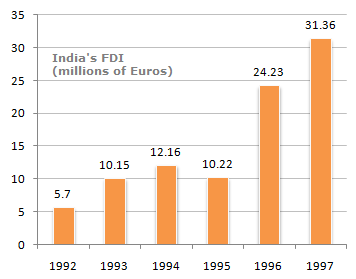# Data Interpretation - Bar Charts - Discussion

The following bar chart shows the trends of foreign direct investments(FDI) into India from all over the world.

Trends of FDI in India3.

If India FDI from OPEC countries was proportionately the same in 1992 and 1997 as the total FDI from all over the world and if the FDI in 1992 from the OPEC countries was Euro 2 million. What was the amount of FDI from the OPEC countries in 1997 ?

 [A]. 11 [B]. 10.72 [C]. 11.28 [D]. 11.5

Explanation:

Let x be the FDI in 1997.

Then: (2/5.7) = (x/31.36)

x = (2/5.7) x 31.36

x = 11

 Naga said: (Mar 15, 2011) Can I have more clear exp please ?

 Avik Mondal said: (Aug 20, 2013) How is 2/5.7 is coming?

 Vishnudas Pachange said: (Jan 10, 2019) (1992)5.7 proportional to (1997) 31.36. It means 31.36/5.7=proportionality constant k --------> (1) Now, x/(2 million) = proportionality constant k -------> (2) Divide equation 1/2. then x=11.

 Sangha said: (Aug 30, 2019) I didn't understand. Please explain to me to get it.